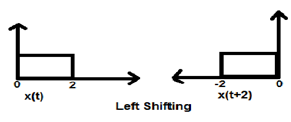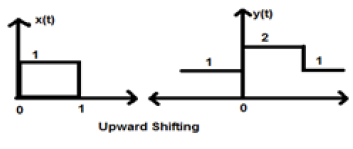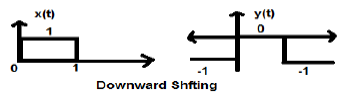# DSP - Operations on Signals Shifting

#### Digital Marketing Online Training

23 Lectures 2 hours

#### How To Start Your Own Digital Marketing Agency

125 Lectures 9 hours

#### Digital Marketing Agency Elite Consultants Masterclass

140 Lectures 20 hours

Shifting means movement of the signal, either in time domain (around Y-axis) or in amplitude domain (around X-axis). Accordingly, we can classify the shifting into two categories named as Time shifting and Amplitude shifting, these are subsequently discussed below.

## Time Shifting

Time shifting means, shifting of signals in the time domain. Mathematically, it can be written as

$$x(t) \rightarrow y(t+k)$$

This K value may be positive or it may be negative. According to the sign of k value, we have two types of shifting named as Right shifting and Left shifting.

### Case 1 (K > 0)

When K is greater than zero, the shifting of the signal takes place towards "left" in the time domain. Therefore, this type of shifting is known as Left Shifting of the signal.

Example### Case 2 (K < 0)

When K is less than zero the shifting of signal takes place towards right in the time domain. Therefore, this type of shifting is known as Right shifting.

Example

The figure given below shows right shifting of a signal by 2.## Amplitude Shifting

Amplitude shifting means shifting of signal in the amplitude domain (around X-axis). Mathematically, it can be represented as −

$$x(t) \rightarrow x(t)+K$$

This K value may be positive or negative. Accordingly, we have two types of amplitude shifting which are subsequently discussed below.

### Case 1 (K > 0)

When K is greater than zero, the shifting of signal takes place towards up in the x-axis. Therefore, this type of shifting is known as upward shifting.

Example

Let us consider a signal x(t) which is given as;

$$x = \begin{cases}0, & t < 0\\1, & 0\leq t\leq 2\\ 0, & t>0\end{cases}$$

Let we have taken K=+1 so new signal can be written as −

$y(t) \rightarrow x(t)+1$ So, y(t) can finally be written as;

$$x(t) = \begin{cases}1, & t < 0\\2, & 0\leq t\leq 2\\ 1, & t>0\end{cases}$$### Case 2 (K < 0)

When K is less than zero shifting of signal takes place towards downward in the X- axis. Therefore, it is called downward shifting of the signal.

Example

Let us consider a signal x(t) which is given as;

$$x(t) = \begin{cases}0, & t < 0\\1, & 0\leq t\leq 2\\ 0, & t>0\end{cases}$$

Let we have taken K = -1 so new signal can be written as;

$y(t)\rightarrow x(t)-1$ So, y(t) can finally be written as;

$$y(t) = \begin{cases}-1, & t < 0\\0, & 0\leq t\leq 2\\ -1, & t>0\end{cases}$$